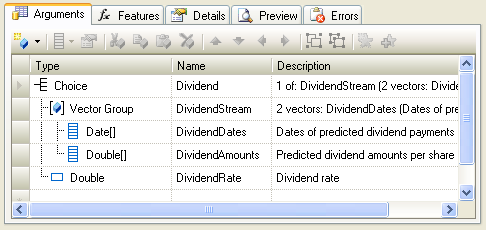XLL+ Class Library (7.0)

# Choice arguments

Groups allow you to tie a set of real arguments to a single Excel argument. Another way to group arguments together is to use a Choice, to transform the Excel input into just one real argument, choosing from a list of mutually exclusive inner arguments. A Choice inspects the Excel input and validates it against each of the arguments held within the choice. As soon as one succeeds, validation stops.

### Example

A simple example is a dividend stream argument. This argument can be either a set of predicted cash-flows - with two columns containing dates and amounts - or a single interest rate.The picture above shows the organization of the Choice group. The outer argument, Dividend, is the argument that is visible in Excel.

When the add-in function validates the input, it first tries to match the the first member of the choice, DividendStream. If it finds that the input consists of two columns, the first containing dates and the second containing numbers, then it reads the values of the columns into the inner arguments, DividendDates and DividendAmounts, and stops processing the argument.

However, if the input does not match DividendStream, then the add-in function tries again, and checks whether the input is a single number, DividendRate. If it matches, then the inner argument DividendRate is set to the value of the input, and processing for the argument is complete.

If the input does not match either of the choice arguments, then an exception of type `CXlChoiceFailedException` is thrown. The add-in function aborts, and an error is returned to Excel:

```#ERROR: Failed to convert Dividend to any of the permitted types
```

### Coding with choices

The validation code generated for the example above is as follows:CopyC++
```CXlOper* ChoiceFn1_Impl(CXlOper& xloResult, const CXlOper* Dividend)
{
// Input buffers
std::vector<long> DividendDates;
std::vector<double> DividendAmounts;
double DividendRate;
// Validate and translate inputs
int Dividend__index = -1;
if (Dividend__index < 0) {
try {
(), L"Dividend", 0, L"DividendDates", XLA_TRUNC_ONEMPTY|
XLA_TRUNC_ONBLANK|XLA_OPER_IS_GROUP);
XlReadGroupedVector(*Dividend, DividendAmounts, L"Dividend", 1,
L"DividendAmounts", XLA_TRUNC_ONEMPTY|XLA_TRUNC_ONBLANK|
XLA_OPER_IS_GROUP);
Dividend__index = 0;
} catch(...) {}
}
if (Dividend__index < 0) {
try {
Dividend__index = 1;
} catch(...) {}
}
if (Dividend__index < 0)
throw CXlChoiceFailedException("Dividend");
// End of generated code
//}}XLP_SRC
// TODO - set the value of xloResult, or return another value
//          using CXlOper::RetXXX() or throw a CXlRuntimeException.
return xloResult.Ret();
}```

You will see that an additional local variable `Dividend__index` has been declared, and initialized to `-1`.

The validation code tries each element of the choice in turn. If validation succeeds, then `Dividend__index` is set to the index of the member that succeeded. If no member passes validation, then `Dividend__index` remains `-1`, and the validation of the entire choice fails.

The table below lists which local variables contain valid values for each value of `Dividend__index`.

Dividend__index Valid inputs
-1 None, and an exception is thrown
0 DividendDates & DividendAmounts
1 DividendRate

Your code can use the value of `Dividend__index` in a switch statement:CopyC++
```switch (Dividend__index)
{
case 0:
xloResult.Format("%lu predicted dividends", DividendDates.size());
break;
case 1:
xloResult.Format("%lf rate", DividendRate);
break;
}```

This approach takes a lot of the complexity associated with multi-format inputs and delegates it to the generated code, leaving your own code simpler to understand and maintain.